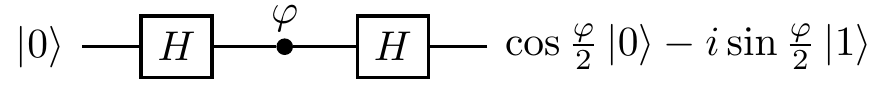## 1.8 Computational complexity

Is there a compelling reason why we should care about quantum computation? It may sound like an extravagant way to compute something that can be computed anyway. Indeed, your standard laptop, given enough time and memory, can simulate pretty much any physical process. In principle, it can also simulate any quantum interference and compute everything that quantum computers can compute. The snag is that this simulation is, in general, very inefficient. And efficiency does matter, especially if you have to wait more than the age of the Universe for your laptop to stop and deliver an answer!31

In order to solve a particular problem, computers (classical or quantum) follow a precise set of instructions called an algorithm. Computer scientists quantify the efficiency of an algorithm according to how rapidly its running time, or the use of memory, increases when it is given ever larger inputs to work on. An algorithm is said to be efficient if the number of elementary operations taken to execute it increases no faster than a polynomial function of the size of the input.32 We take the input size to be the total number of binary digits (bits) needed to specify the input. For example, using the algorithm taught in elementary school, one can multiply two n digit numbers in a time that grows like the number of digits squared, n^2. In contrast, the fastest-known method for the reverse operation — factoring an n-digit integer into prime numbers — takes a time that grows exponentially, roughly as 2^n. This is considered inefficient.The class of problems that can be solved in polynomial time by a deterministic computer is represented by the capital letter \texttt{P}, for polynomial time. The class of problems that can be solved in polynomial time by a probabilistic computer is called \texttt{BPP}, for bounded-error probabilistic polynomial time.

It is clear that \texttt{BPP} contains \texttt{P}, since deterministic computation is a special case of probabilistic computation in which we never consult the source of randomness. When we run a probabilistic (a.k.a. randomised) computation many times on the same input, we will not get the same answer every time, but the computation is useful if the probability of getting the right answer is high enough.

Finally, the class of problems that can be solved in polynomial time by a quantum computer is called \texttt{BQP}, for bounded-error quantum polynomial. Since a quantum computer can easily generate random bits and simulate a probabilistic classical computer, \texttt{BQP} certainly contains the class \texttt{BPP} (which itself contains the class \texttt{P}). Here we are interested in problems that are in \texttt{BQP} but not known to be in \texttt{BPP}. The most popular example of such a problem is factoring.A quantum algorithm, discovered by Peter Shor in 1994, can factor n-digit numbers in a number of steps that grows only as n^2, as opposed to the 2^n that we have classically.33 Since the intractability of factorisation underpins the security of many methods of encryption, Shor’s algorithm was soon hailed as the first “killer application” for quantum computation: something very useful that only a quantum computer could do. Since then, the hunt has been on for interesting things for quantum computers to do, and at the same time, for the scientific and technological advances that could allow us to build quantum computers in reality.

1. The age of the Universe is currently estimated at 13.772 billion years.↩︎

2. Note that technological progress alone, such as increasing the speed of classical computers, will never turn an inefficient (exponential scaling) algorithm into an efficient (polynomial scaling) algorithm. Why?↩︎

3. It must be stressed that not all quantum algorithms are so efficient. In fact many are no faster than their classical counterparts. Which particular problems will lend themselves to quantum speed-ups is an open question.↩︎